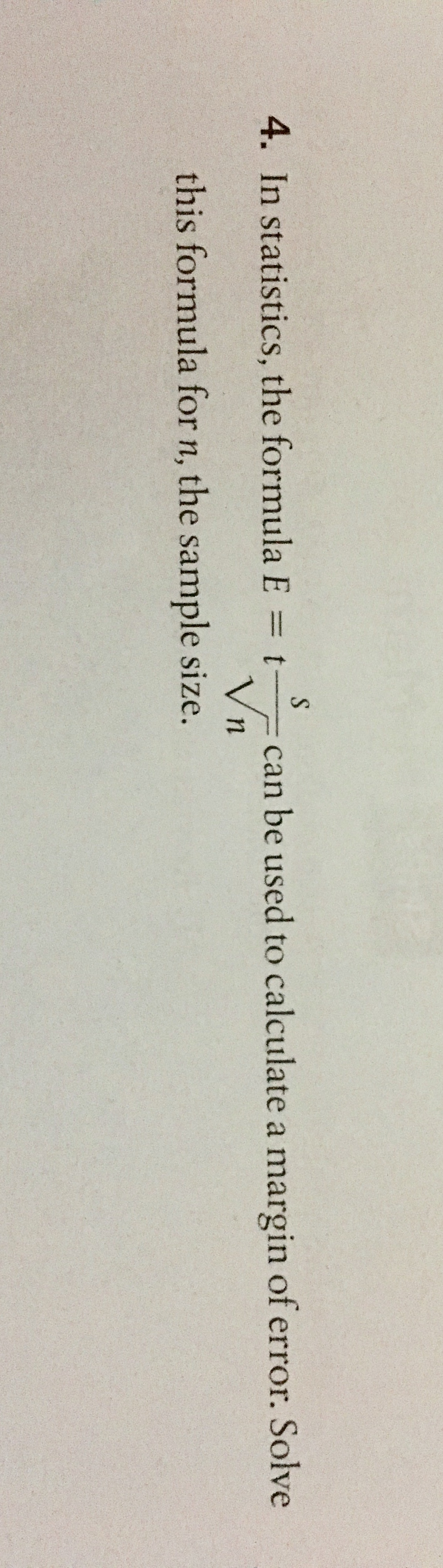# 4. In statistics, the formula E = tcan be used to calculate a margin of error. Solvethis formula for n, the sample size.

Questionhelp_outlineImage Transcriptionclose4. In statistics, the formula E = t can be used to calculate a margin of error. Solve this formula for n, the sample size. fullscreen
check_circle

Step 1

This exercise concerns changing the subject of the equation from E (error) to n( the sample size)

Step 2

The given equation gives the formula for E (error) in terms of the variables, t, s and n (sample size). Required to express n in terms of E, s and t.

Step 3

### Want to see the full answer?

See Solution

#### Want to see this answer and more?

Solutions are written by subject experts who are available 24/7. Questions are typically answered within 1 hour.*

See Solution
*Response times may vary by subject and question.
Tagged in

### Equations and In-equations